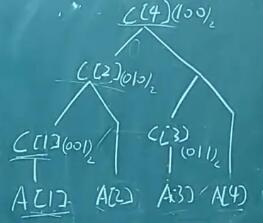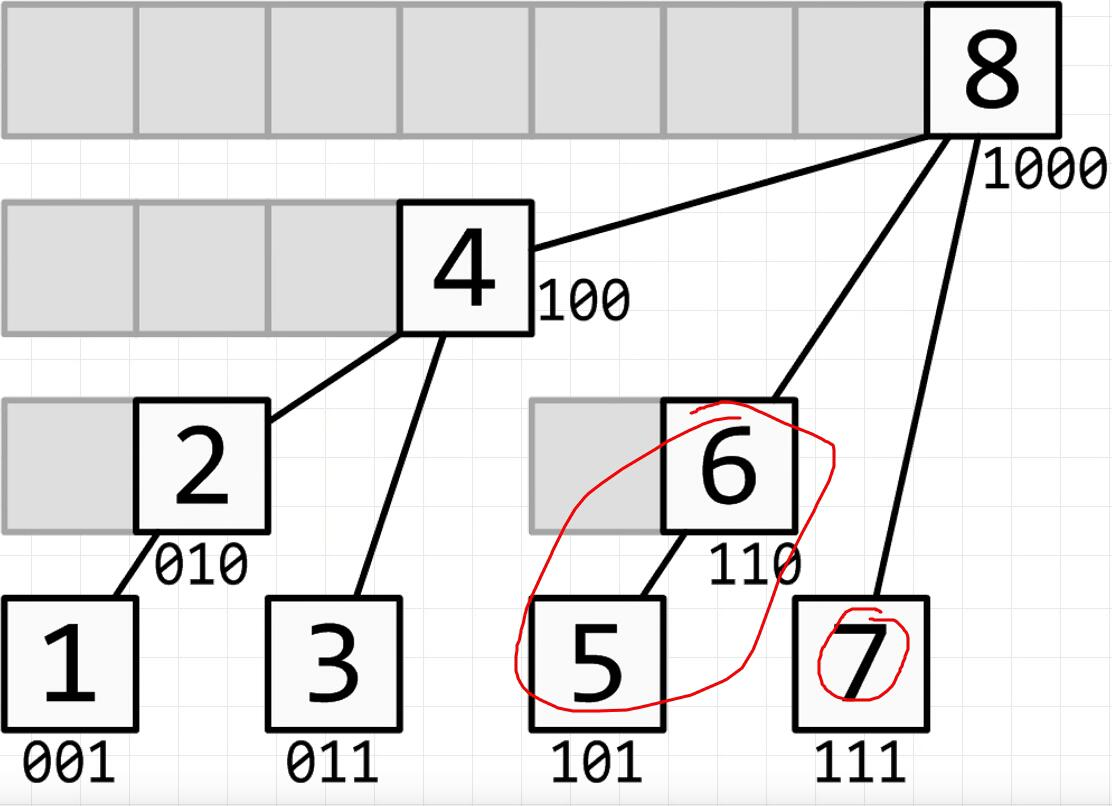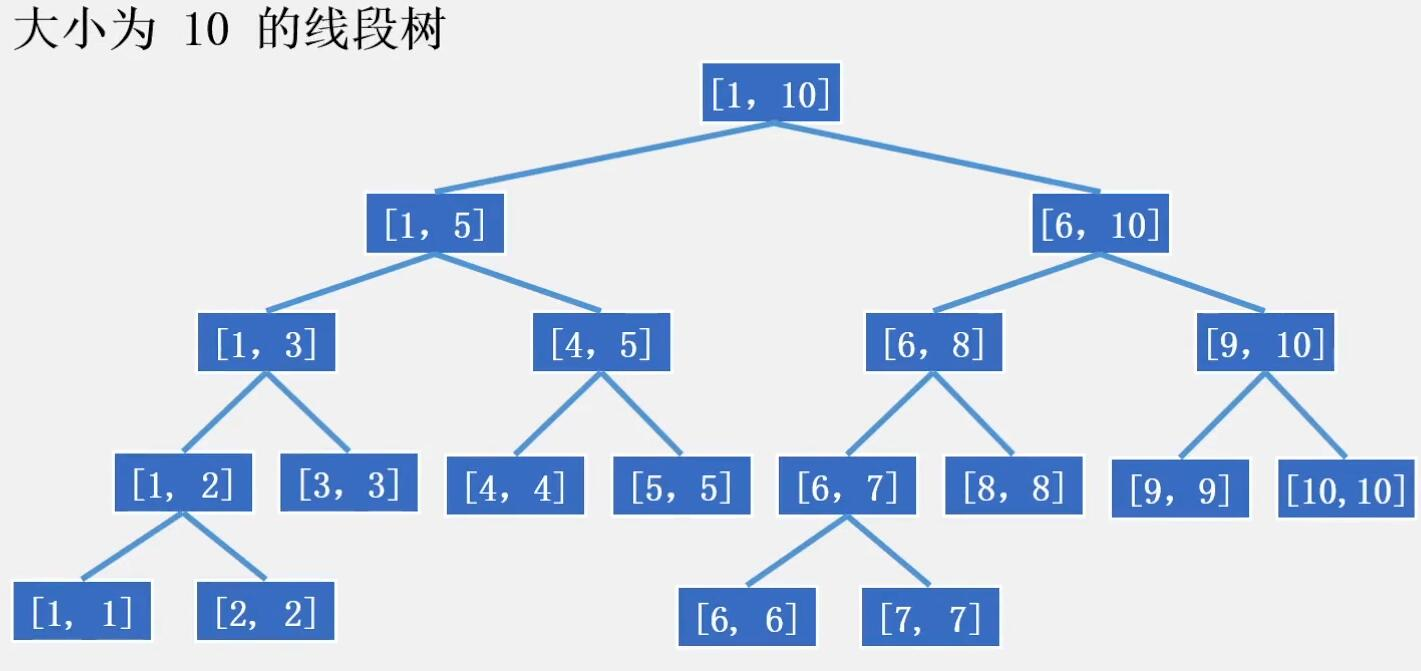Mrli

Contacts:
QQ博客园

# ACM-树状数组和线段树

2019/12/08
Word count: 2,650 | Reading time: 12min

Q：需求：（老板给你分了台2GHz单核，内存500M的服务器然后让你写程序）程序每秒会收到一组数据，每组数据包含10万条命令，总共会有100万个仓库，每个仓库库存没有上限且可为负，库存初始为0。你需要在一秒内完成全部的命令，然后将查询结果按顺序得出后传回，命令如下：

• Add i j，i和j为正整数，表示第i个仓库增加j个库存（j不超过220）
• Sub i j，i和j为正整数，表示第i个仓库减少个库存（j不超过220）
• Query i j，i和j为正整数，i<=j，表示询问第i到第个仓库的总库存；End 表示结束，这条命令在每组数据最后出现；

# 树状数组

## low bit算法

Q： 6可以怎么计算得到呢？
A： 6==0110，i=110，通过lowbit可以拆分成两个数100+010=110

①S+= C
②找到i最右边的1，即得到$lowbit=(10)_{2}=(2)_{10}$
③更新i:$i'= i - lowbit = (0110)_{2}-(0010)_{2}=(0100)_{2}$，即$i'=6-2=(4)_{10}$
④S+= C
⑤再继续找i最右边的1，即得到了$lowbit=(100)_{2}=(4)_{10}$
⑥更新i:$i'= i - lowbit =(0100)_{2}-(0100)_{2}=(0000)_{2}$
⑦此时i最右边无1(即全零为0)，算法结束，即最终C=C + C

## 计算步骤：

1. 找到$(n)_2$最右边的1的数$(n1)_2$
2. 更新$n'=(n)_2 - (n1)_2$
3. 如果$(n')_2$还能找到1，那么重复1-2

1. Ai：第i个仓库的库存数

2. Ci：所构建的数的第i个节点的值，C[i] = A[i-lowbit(i)+1] + …+A[i]

3. Si：第1个仓库到第i个仓库库存数之和

4. Ans（i，j）：显然就等于$S_{R}-S_{L-1}$

## 形式

▲lowbit又叫子叶数，表示了有多少个A[]相加，C[i]表示从C[i] = A[i-lowbit(i)+1] + …+A[i] (<===>C[i] = A[i-2^x+1] + … + A[i])，

For Example： i=6## 参考：# 线段树

## 特点：

• 每个节点维护一个闭区间1,r的信息。
• 根节点表示[1,n]的信息。如果1=r那就是叶子结点.
• 如果1<r那就是内部节点,它有两个子节点[1,(1+r)/2]，[(1+r)/2+1,r].

## 性质

• 节点数：假设线段树处理的数列长度为n，即根节点的区间为[1，n].那么结点数不超过2n个.因此线段树的空间复杂度是0（n）。是完满二叉树(Full Binary Tree)，但一般申请4*n的空间大小
• 深度：可以看作满二叉树，深度不超过log2(n-1)+1
• 线段树能把区间上的任意一条长度为L的线段都分成不超过2*log(L)条线段。

• 由于链表表示的内存是不连续的，且申请指针比较费时间，所以采用数组矩阵的形式存储。
• 数组存储也有一点不好，因为线段树并不是真正的完全二叉树。
最后一层可能很空。且空节点的数量可以达到2n个。
因此维护长度为n的序列，用数组存线段树的话，最好要开到4*n的长度，才能保证数组不越界。

## 形式Q：对于第i个数，我们要统计前面有多少个数大于a[i]。

e.g.如果一个值为1，一个值为108，那么要开108大小

Solve: 10^9范围太大了，因此我们先要对n个数进行离散化。离散化的过程，就是对n个数进行排序，最小的数赋值为1第二小的赋值为2，以此类推，这样n个数的取值范围就在[1，n]中了。

e.g.上面的情况，将会把1–>1,10^8–>2,那么只要开长度为2的线段树就够了

SWPU-ACM每周算法讲堂-线段树入门（一）

Author: Mrli